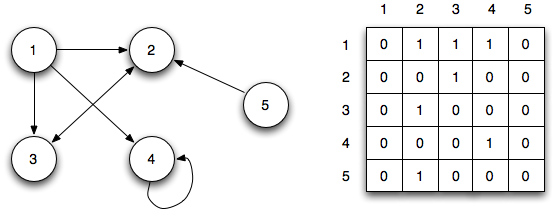While a vector is a list of numbers that depend on only one index, the most direct interpretation of a matrix is a table of numbers that depend on two indices, $$i$$, and $$j$$. In this case, the rows and columns of the matrix usually have some simple interpretation. Here are some examples.

Images. A black and white image with $$M \times N$$ pixels is naturally represented as an $$M \times N$$ matrix.

Rainfall Data. An $$m \times n$$ matrix $$A$$ gives the rainfall at $$m$$ different locations on $$n$$ consecutive days, so $$A_{42}$$ is the rainfall at location 4 on day 2.

Asset Returns. A $$T \times n$$ matrix $$R$$ gives the returns of a collection of $$n$$ assets (the universe of assets) over $$T$$ periods (it’s a time series), with $$R_{ij}$$ giving the return of asset $$j$$ in period $$i$$. So $$R_{12, 7} = -0.03$$ means that asset 7 had a 3% loss in period 12.

Supplier Prices. An $$m \times n$$ matrix $$P$$ gives the prices of $$n$$ different goods from $$m$$ different suppliers. $$P_{ij}$$ is the price that supplier $$i$$ changes for good $$j$$.

Customer Purchase History. An $$n \times N$$ matrix $$P$$ can be used to store a set of $$N$$ customers’ purchase histories of $$n$$ products, items, or services, over some period.

## Matrix Representation of a Collection of Vectors

If $$x_i, \cdots, x_N$$ are $$n$$-vectors that give the $$n$$ feature values for each of $$N$$ objects, we can collect them into one $$n \times N$$ matrix often called a data matrix or feature matrix.

$X = [ \ x_1 \ x_2 \ \cdots \ x_N \ ]$

## Matrix Representation of a Relation or Graph

Suppose we have $$n$$ objects labeled $$1, \cdots, n$$. A relation $$\mathcal{R}$$ on the set of objects $${1, \cdots, n}$$ is a subset of ordered pairs of objects. $$\mathcal{R}$$ could represent a preference relation among $$n$$ possible products or choices, with $$(i, j) \in \mathcal{R}$$ meaning that choice $$i$$ is preferred to choice $$j$$. A relation can also be viewed as a directed graph, with nodes labeled $$1, \cdots, n$$ and a directed edge from $$j$$ to $$i$$ for each $$(i, j) \in \mathcal{R}$$. This is typically drawn as a graph, with arrows indicating the direction of the edge. Normally, a relation is represented by the $$n \times n$$ matrix $$A$$ with

$A_{ij} = \begin{cases} 1 & (i, j) \in \mathcal{R} \\ 0 & (i, j) \notin \mathcal{R} \end{cases}$